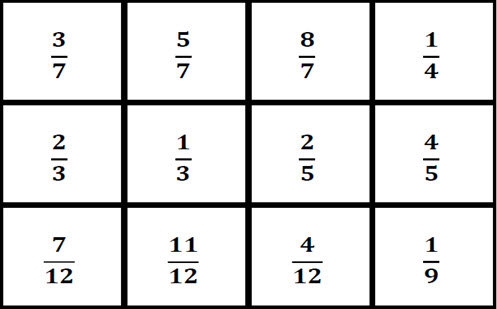Go to website

# reSolve: Fraction Benchmarks

This sequence of two lessons focuses on the idea that fractions are numbers, and builds students' understanding of the relative sizes of fractions. Students build their fractional sense through determining if fractions are closest to 0, 1/2 or 1. In the first lesson, students use sets of fraction cards to sort fractions in numerical order and decide whether they are closer to 0, 1/2 or 1. In the second lesson, students build on this understanding to play a game and develop further strategies for ordering fractions. The lessons are outlined in detail including curriculum links, vocabulary, materials needed, sample answers, discussion points and student resources. This sequence is part of the reSolve: Mathematics by Inquiry program.

Year level(s) Year 6
Audience Teacher
Purpose Teaching resource
Teaching strategies and pedagogical approaches Mathematics investigation
Keywords number lines, fractions, related denominators

## Curriculum alignment

Curriculum connections Numeracy
Strand and focus Number, Build understanding, Apply understanding
Topics Fractions
AC: Mathematics (V9.0) content descriptions
AC9M6N03
Apply knowledge of equivalence to compare, order and represent common fractions including halves, thirds and quarters on the same number line and justify their order

Numeracy progression Interpreting fractions (P7)